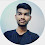# Staircase Hackerrank Solution in C | Algorithm Solutions

We are going to find the hackerrank solutions of the algorithms section and try to solve the staircase hackerrank solution in c with full code and logic explanation. In this solution, we are also going to add the output of the code so that our reader can understand that our staircase hackerrank solution is working fine and solutions are updated timely.

Staircase detail

This is a staircase of size n = 4:

#
##
###
####

Its base and height are both equal to n. It is drawn using # symbols and spaces. The last line is not preceded by any spaces.

Write a program that prints a staircase of size n.

Function Description

Complete the staircase function in the editor below.

staircase has the following parameter(s):

int n: an integer

Print

Print a staircase as described above.

Input Format

A single integer, n, denotes the size of the staircase.

Constraints

0 < n <= 100.

Output Format

Print a staircase of size n using # symbols and spaces.

Note: The last line must have 0 spaces in it.

Sample Input

Sample Output

#
##
###
####
#####
######

Explanation

The staircase is right-aligned, composed of # symbols and spaces, and has a height and width of n = 6.

## Staircase Hackerrank Solution in C

``````#include <stdio.h>

int main()
{
int n, i, j, s = 0, pattern = 0;
scanf("%d", &n);
/*
No of rows of pattern
*/
for (i = 0; i < n; i++)
{
s = n - (i + 1);
for (j = 0; j < s; j++)
{
printf(" ");
}

/*We are going to print no. of space minus 1 each time */
pattern = i + 1;

for (j = 0; j < pattern; j++)
{
printf("#");
}

/*
Printing the Hash("#") pattern
*/
printf("\n");
}
}``````

### Staircase Hackerrank Solution Logic

Let's dive to find the solution. Our challenge is to find the Staircase Program in C Hackerrank So lets Do it. First, we have to understand the problem statement then only we can write code in our desired programming language.

Staircase Program in C Hackerrank there is no logic we have to just print the pattern in a staircase by using the hash (#) symbol. we always use stairs in our daily life now turn to implement them in programming life. See the below pattern this is called a staircase. When asking for the user to enter the number that means the height of the staircase. In this case, I am taking Height = 10.

### Similar to Staircase

Previous Post
Next Post

#### post written by: Ghanendra Yadav

Hi, I’m Ghanendra Yadav, SEO Expert, Professional Blogger, Programmer, and UI Developer. Get a Solution of More Than 500+ Programming Problems, and Practice All Programs in C, C++, and Java Languages. Get a Competitive Website Solution also Ie. Hackerrank Solutions and Geeksforgeeks Solutions. If You Are Interested to Learn a C Programming Language and You Don't Have Experience in Any Programming, You Should Start with a C Programming Language, Read: List of Format Specifiers in C.

1.how is it possible

1.Why it's not possible, here simply firstly we are leaving space less the input number and increasing a loop by one and also decreasing a space count by one and print the symbol two time and so on. It's easy to try again I am sure you will catch the logic easy.

Thanks

2.Thank you very much.
I managed to modify my code quoting your logic.
However, I don't understand logic for the space part.

s = n - (i+1)

so in your code for space
i < n
j < s

so I try to sub n with some number
if n = 1, i = 0, j = 0;
n = 2, i = 1, j = 0;
n = 3, i = 2, j = 0;
etc

so i is representing column?
j is always 0, does this line of code
s = n - (i+1);
for(j = 0; j < s; j++)
necessary?

I am a beginner.
Thank you very much for your help.

3.